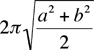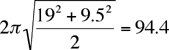Quandaries and Queries I have an oval pool of which I am trying to find the circumference.  it is 38 feet long and 19 feet wide  thank you Hi John, I am going to interpret oval to mean ellipse. In this case there is no simple formula for the circumference. An expression in terms of an integral can be found in our answer to a previous question. There is however a simple approximation to the circumference given bywhere a and b are the semi-axis lengths. Your semi-axis lengths are 19 feet and 9 1/2 feet and hence the approximation I get for the perimeter isfeet. Penny Go to Math Central